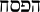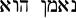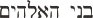# 153 is a number in love with itself – Narcissistic number

Did you know that a number can be in love with itself?

Can numbers be narcissistic? As a matter of fact, yes they can be.

What is a narcissistic number? It is a number that is the sum of its own digits when each digit is raised to the power of the number of digits.

There is a deep link between the symbol of Christ as the Fish/Fisherman, the Sacrifice, the Passover (153), the Covenant (612= 4 x 153), and the Net (1224 = 2 x 612) in John 21:11 that caught the 153 fish. The Number 153 is the seventeeth triangular number, hence also is the sum of the integers from 1 to 17:

(17) = 1 + 2 + … + 16 + 17 = 153The Number 153 – I am the Lord Thy God

### Isaiah 43:1-3

King James Version (KJV)

43 For I am the Lord thy God, the Holy One of Israel, thy Saviour: I gave Egypt for thy ransom, Ethiopia and Seba for thee.

### John 21:11

King James Version (KJV)

11 Simon Peter went up, and drew the net to land full of great fishes, an hundred and fifty and three: and for all there were so many, yet was not the net broken.

### John 10

King James Version (KJV)

10 Verily, verily, I say unto you, He that entereth not by the door into the sheepfold, but climbeth up some other way, the same is a thief and a robber.

But he that entereth in by the door is the shepherd of the sheep.

 The Number 153 I am the Lord thy GodAni YHVH Elohikah The PassoverHaPesach He is faithful [Num 12.7]Hua Nameen He shall cause to inherit [Deut 1.38]yinchilena Sons of God [Gen 6.4]Beni HaElohim Side, Part [John 21.6]merai

The Number 153 appears explicitly in John 21.10, and implicitly in the values of the words used (side = 153, net = 1224). It has many interesting properties. For example:

153 = 1 + 2 + 3 + … + 16 + 17 = Sum(17)

153 = 1! + 2! + 3! + 4! + 5!

153 = 13 + 53 + 33

# The Fish Created on the 5th Day

References about number 153 can be found in the New Testament, where in the net Simon Peter drew from the Sea of Tiberias held 153 fishes. Some of the interesting properties of number 153 published in  are:

Curious properties of number 153:

• It is the smallest number which can be expressed as the sum of cubes of its digits:

153 = 1+ 5+ 33

• It is equal to the sum of factorials of number from 1 to 5:

153 = 1! + 2! + 3! + 4! + 5!

• The sum of digits of 153 is a perfect square:

1 + 5 + 3 = 9 = 32

• The sum of aliquot divisors of 153 is also a perfect square:

1 + 3 + 9 + 17 + 51 = 81 = 92

Aliquot divisors of a number are all the divisors of that number excluding the number itself but including 1. It is seen that the sum of aliquot divisors of 153 is the square of the sum of the digits of 153.

• On adding the number 153 to its reverse,504 is obtained, whose square is the smallest square which can be expressed as the product of two different non-square numbers which are reverse of one another:

153 + 351 = 504

5042 = 288 x 882

• It can be expressed as the sum of all integers from 1 to 17. In other words, 153 is the 17th triangular number. Since reverse of 153, i.e. 351 is also a triangular number, 153 can be termed as a reversible triangular number.
• Number 153 is also a Harshad number(also called Niven number), i.e. it is divisible by the sum of its own digits:

153 / (1 + 5 + 3) = 17

Since reverse of 153, i.e. 351 is also a Harshad number(or Niven Number), 153 can be termed as a reversible Harshad number(or reversible Niven Number).

• It can be expressed as the product of two numbers formed from its own digits:

153 = 3 * 51

Note that the digits used in multipliers are same as in product.

• The number 135, which is formed by rearranging the digits of 153, can be expressed as:

135 = 11 + 32 + 53

• The sum of all divisors of 153 is 234.

1 + 3 + 9 + 17 + 51 + 153 = 234

The product of aliquot divisors of 153 is 23409:

1 * 3 * 9 * 17 * 51 = 23409

Note that the product of aliquot divisors of 153 contain the sum of all divisors of 153 juxtaposed:

23409 = 234:09

234 = Sum of all divisors of 153

09 = Square root of the sum of aliquot divisors of 153.

• When the cubes of the digits of any number, that is, a multiple of 3 are added, and then this process is repeated, the final result is 153, where the process ends because 153 = 13 + 5+ 33

For example: Take the number 108

13 + 03 + 83 = 513 and 53 + 1+ 33 = 153

So, the number 108 reaches 153 in two cycles and it can be represented as

108®513®153

A detailed study of all numbers up to 105reveals that all numbers which are multiple of 3 and are less than 105 reach 153 (after the repeated process of summing the cubes of digits is done) in maximum 14 cycles. However, maximum 13 cycles is required for all numbers, which are multiple of 3 and are less than 10,000.

The smallest number, which requires 13 cycles to reach 153, is 177, i.e.,

177® 687® 1071®345® 216® 225® 141® 66® 432® 99® 1458® 702® 351® 153

Table 1 indicates the smallest numbers, which reach 153 in cycles from 1 to 14.

TABLE: 1

 No. of cycles Smallest number 1 135 2 18 3 3 4 9 5 12 6 33 7 114 8 78 9 126 10 6 11 117 12 669 13 177 14 12558

It may be noted that the smallest number to reach 153 in 15 cycles will be more than 1019. Can you find it?

Some New Observations on number 153:

• The smallest number to reach 153 in 16 cycles will be more than 1061042524005486968, so not advisable to try it. But you can certainly try to find the smallest number reaching 153 in 15 cycles and check your answer by clicking here.
• 10 + 51 + 32 = 1 * 5 * 3
• If p(x) represents the number of primes up to x, then the following holds good:

p (153) = p (15) * 3!

• For a beautiful fascinating observation in reciprocal of 153 by Patrick De Geest, click here.
• 10 + 51 + 32 = 15
• 11 + 52 + 33 = 53
• 153153 is the smallest odd abundant number ending in 3.(added on 31-12-2001)
• 153153, 351351, 513513 are all odd abundant numbers.(added on 31-12-2001)
• One of our books of sacred writings is titled The Hidden Words. It is a collection of short meditations, divided into two sections. The first section contains 71 items that were revealed in Arabic; the second section of 82 items was revealed in Persian (Farsi). I think you see my point already….. 71 + 82 = 153. I have often told my fellow believers about this connection between math and our Faith’s literature. They are always quite impressed, to say the least.(Sent by Terry Trotter by email dt 25th Dec, 2001)
• Let us say 153 increasingly from left to right:

1, 15, 153

We find that 115153 is prime?(Sent by G.L.Honaker,Jr. by email dt 3rd Feb, 2002)

• The square root of 153 (i.e. 12.369) is the amount of full moons in one year. (Sent by James Furia by email dt 16th June, 2009)
• The length of the grand gallery inside the Great Pyramid is 153 feet. (Sent by James Furia by email dt 16th June, 2009)
• Binary presentation i.e. 10011001 and hexadecimal presentation i.e. 99 of 153 are palindromes. (Sent by Lauri.Kukko@ilkka-yhtyma.fi by email dt 29th May, 2010)
• Within the Great Pyramid, from the King’s Chamber floor up to the summit platform there are 153 courses of masonry (From the Pyramidology Book #1 by Adam Rutherford). (Sent by Joe Biddy by email dt 1st October, 2010)
• (11 + 55 + 33) mod 1000 = 153 (Sent by M Sihabudin, from Indonesia)
• If you add the highest and lowest number from these six
135 + 531
153 + 513
315 + 351

you will get 666 – an important number in the book of revelation. (Sent by Bjorn Jonasson from Sweden by email dated 01 Jul 2011)
• 153 is the smallest number k, such k4could be split into two primes in two different ways:
1534 = 547981281 and 547981281 can be split into :
5479 , 81281 and 5 , 47981281 (Sent by Claudio Meller by email dated 26 May, 2012)

Curious Properties of 153, Shyam Sunder Gupta, Science Reporter, February 1991, India

Advertisements